Next: Propagation in a dielectric Up: Electromagnetic radiation Previous: Thompson scattering

## Rayleigh scattering

Let us now consider the scattering of electromagnetic radiation by neutral atoms. For instance, consider a hydrogen atom. The atom consists of a light electron and a massive proton. As we have seen, the electron scatters radiation much more strongly than the proton, so let us concentrate on the response of the electron to an incident electromagnetic wave. Suppose that the wave electric field is again polarized in the-direction, and is given by Eq (1120). We can approximate the electron's equation of motion as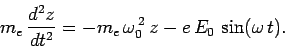(1132)

Here, the second term on the right-hand side represents the perturbing force due to the electromagnetic wave, whereas the first term represents the (linearized) force of electrostatic attraction between the electron and the proton. Indeed, we are very crudely modeling our hydrogen atom as a simple harmonic oscillator of natural frequency. We can think ofas the typical frequency of electromagnetic radiation emitted by the atom after it is transiently disturbed. In other words, in our model,should match the frequency of one of the spectral lines of hydrogen. More generally, we can extend the above model to deal with just about any type of atom, provided that we setto the frequency of a spectral line.

We can easily solve Eq. (1132) to give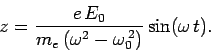(1133)

Hence, the dipole moment of the electron takes the form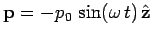, where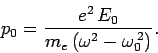(1134)

It follows, by analogy with the analysis in the previous section, that the differential and total scattering cross-sections of our model atom take the form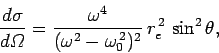(1135)

and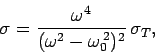(1136)

respectively.

In the limit in which the frequency of the incident radiation is much greater than the natural frequency of the atom, Eqs. (1135) and (1136) reduce to the previously obtained expressions for scattering by a free electron. In other words, an electron in an atom acts very much like a free electron as far as high frequency radiation is concerned. In the opposite limit, in which the frequency of the incident radiation is much less than the natural frequency of the atom, Eqs. (1135) and (1136) yield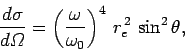(1137)

and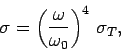(1138)

respectively. This type of scattering is called Rayleigh scattering. There are two features of Rayleigh scattering which are worth noting. First of all, it is much weaker than Thompson scattering (since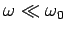). Secondly, unlike Thompson scattering, it is highly frequency dependent. Indeed, it is clear, from the above formulae, that high frequency (short wave-length) radiation is scattered far more effectively than low frequency (long wave-length) radiation.

The most common example of Rayleigh scattering is the scattering of visible radiation from the Sun by neutral atoms (mostly Nitrogen and Oxygen) in the upper atmosphere. The frequency of visible radiation is much less than the typical emission frequencies of a Nitrogen or Oxygen atom (which lie in the ultra-violet band), so it is certainly the case that. When the Sun is low in the sky, radiation from it has to traverse a comparatively long path through the atmosphere before reaching us. Under these circumstances, the scattering of direct solar light by neutral atoms in the atmosphere becomes noticeable (it is not noticeable when the Sun is high is the sky, and radiation from it consequently only has to traverse a relatively short path through the atmosphere before reaching us). According to Eq. (1138), blue light is scattered slightly more strongly than red light (since blue light has a slightly higher frequency than red light). Hence, when the Sun is low in the sky, it appears less bright, due to atmospheric scattering. However, it also appears redder than normal, because more blue light than red light is scattered out of the solar light-rays, leaving an excess of red light. Likewise, when we look up at the sky, it does not appear black (like the sky on the Moon) because of light from solar radiation which grazes the atmosphere being scattered downward towards the surface of the Earth. Again, since blue light is scattered more effectively than red light, there is an excess of blue light scattered downward, and so the sky appears blue.

Light from the Sun is unpolarized. However, when it is scattered it becomes polarized, because light is scattered preferentially in some directions rather than others. Consider a light-ray from the Sun which grazes the Earth's atmosphere. The light-ray contains light which is polarized such that the electric field is vertical to the ground, and light which is polarized such that the electric field is horizontal to the ground (and perpendicular to the path of the light-ray), in equal amounts. However, due to the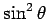factor in the dipole emission formula (1119) (where, in this case,is the angle between the direction of the wave electric field and the direction of scattering), very little light is scattered downward from the vertically polarized light compared to the horizontally polarized light. Moreover, the light scattered from the horizontally polarization is such that its electric field is preferentially perpendicular, rather than parallel, to the direction of propagation of the solar light-ray (i.e., the direction to the Sun). Consequently, the blue light from the sky is preferentially polarized in a direction perpendicular to the direction to the Sun.Next: Propagation in a dielectric Up: Electromagnetic radiation Previous: Thompson scattering
Richard Fitzpatrick 2006-02-02